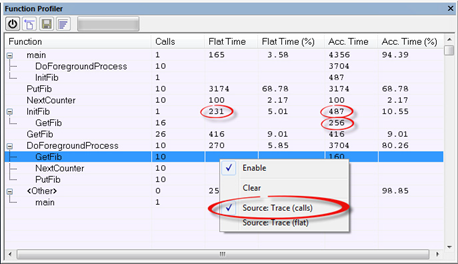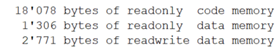﻿ 如何编写有利于编译器优化的代码-《单片机与嵌入式系统应用》杂志社

# 如何编写有利于编译器优化的代码

2021年11月09日 16:02

for(i=0;i!=n;++i)

{

a[i]=b[i];

}

char*buf

voidclear_buf()

{

inti;

for(i=0;i<128;++i)

{

buf[i]=0;

}

}

voidclear_buf(char*buf)

{

inti;

for(i=0;i<128;++i)

{

buf[i]=0;

}

}

foo=a[i++];

foo=a[i];

i=i+1;

i=0;

while(a[i++]!=0)

{

...

}

loop:

temp=i;/*保存操作数的值*/

i=temp+1;/*递增操作数*/

if(a[temp]==0)/*使用保存的值*/

gotono_loop;

...

gotoloop;

no_loop:

loop:

temp=a[i];/*使用操作数的值*/

i=i+1;/*递增操作数*/

if(temp==0)

gotono_loop;

...

gotoloop;

no_loop:

i=0;

while(a[i]!=0)

{

++i;

...

}

loop:

if(a[i]==0)

gotono_loop;

i=i+1;

...

gotoloop;

no_loop: